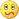# Momentum indicator - page 16186

Smoothed momentum : smoothed_momentum.ex4

____________________

PS: in the example picture, upper is this one, lower is regul;ar (built in) momentum. To turn the smoothing off, set the smoothing to <= 1255627

In order to prevent a lot of false signals momentum indicators usually trigger, a very low lag smoothing is applied to momentum in order to filter out false signals.

____________________

PS: not to be confused with velocity indicator (which is also a momentum indicator but the calculation is completely different from momentum indicator and which produces much smoother results than the momentum indicator)186

Momentum can be a useful indicator when it comes to trend finding, but, as far as I am concerned, it has a problem of too much signals when it is around some levels. Simple smoothing does not work (adds lag) neither using average instead of "raw price" (for same reason - adds lag). This is one possible way to overcome that problems

Instead of calculating just a single value of momentum this one is cumulating all the momentum values up to length desired (so it is mom)1_+mom(2)+mom(3)+...+mom(n)) and you can use an averaged (smoothed) values for calculation and in this case the surprise is that there is no lag added compared to "regular" momentum calculation (in a lot of cases quite the opposite - seems that cumulating values lessens the lag). Here is one comparison of the cumulative calculation (upper) and "regular" momentum (lower)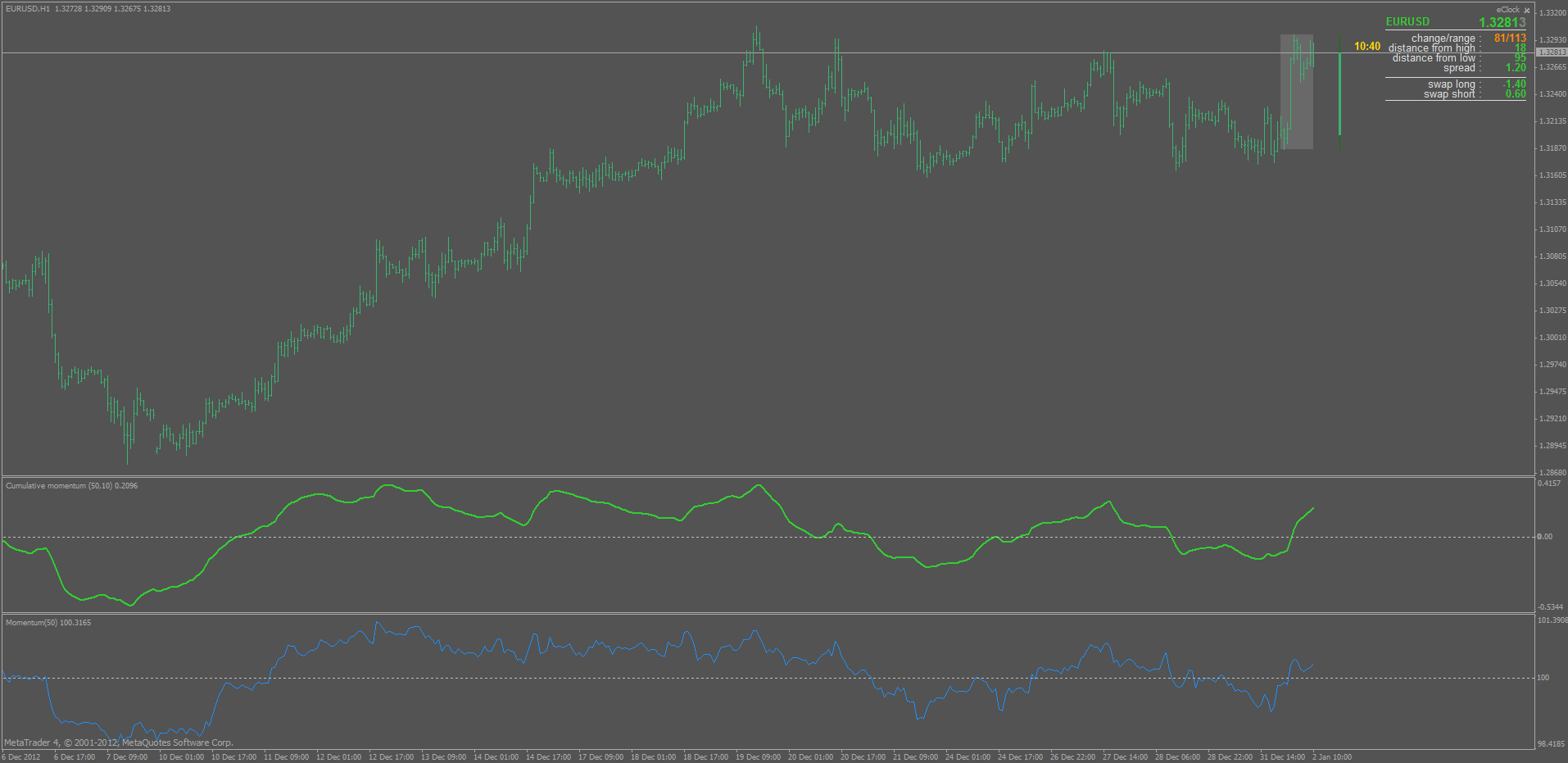PS: it should not be confused with a "momentum of average" (or a "slope of average)). Here is a comparison of the 3 : upper is the cumulative momentum, middle is the regular, and lowest is the slope (momentum) of average :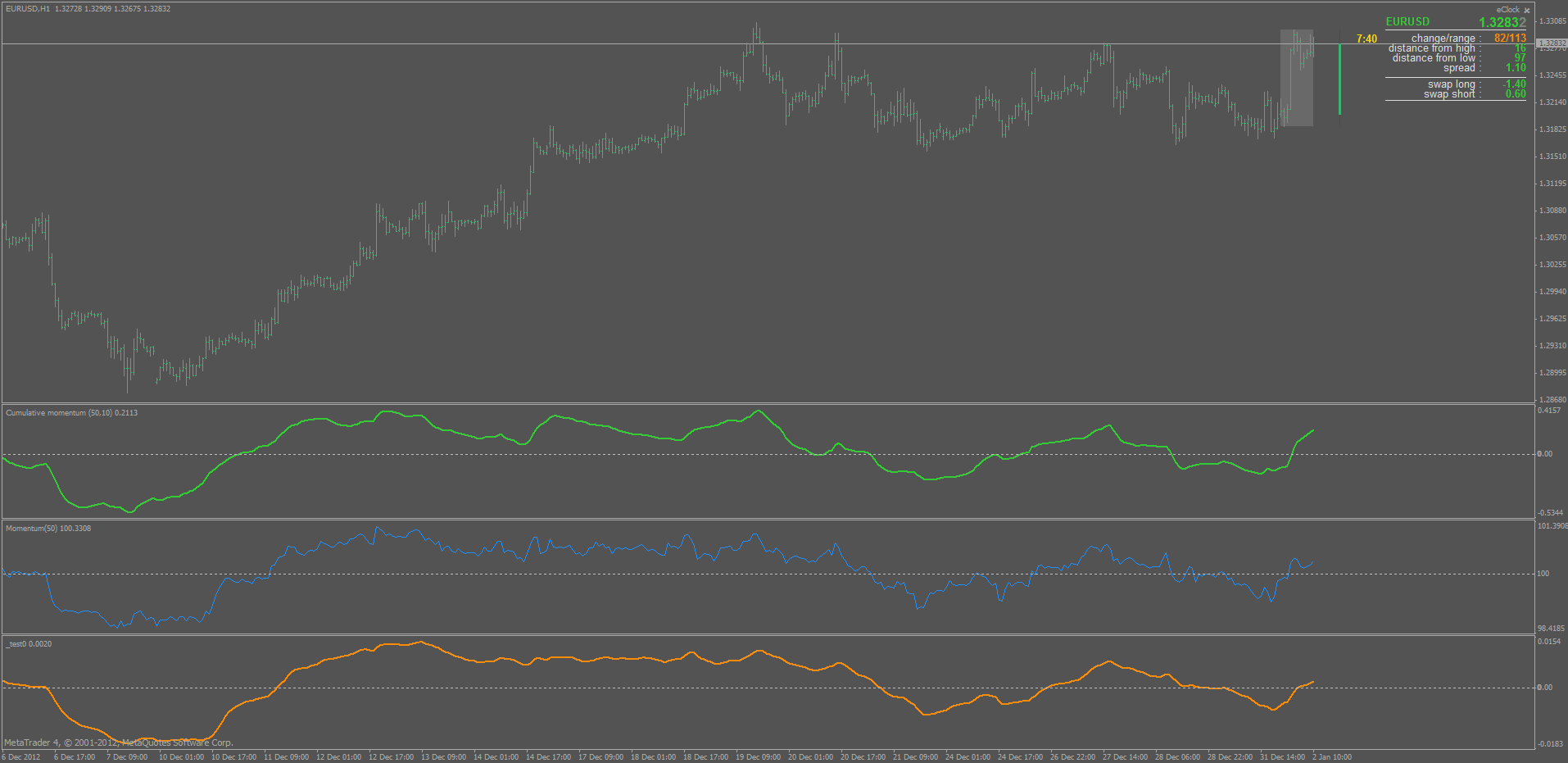As it is obvious, slope (momentum) of average adds lag (as it would some "post smoothing" of momentum do that too)9625

Tradersm, working on build 840 for me.

Files:
cm.png  58 kb977

mrtools:
Tradersm, working on build 840 for me.

Hi Mrtools

What was the point arrows (in the main windows) belong to?

Thanks9625

talaate:
Hi Mrtools

What was the point arrows (in the main windows) belong to?

Thanks

Talaat E, It's Brain Trend signal from here: https://www.mql5.com/en/forum/183798/page168186

A very, very simple way to calculate velocity (what is sometimes called a Jurik velocity) Just to remind that velocity is in fact a smoothed momentum which can be very helpfull since momentum tends to give some false signals due to its "rough" values calculated. Here is one comparison of the momentum (blue line) and velocity (red line).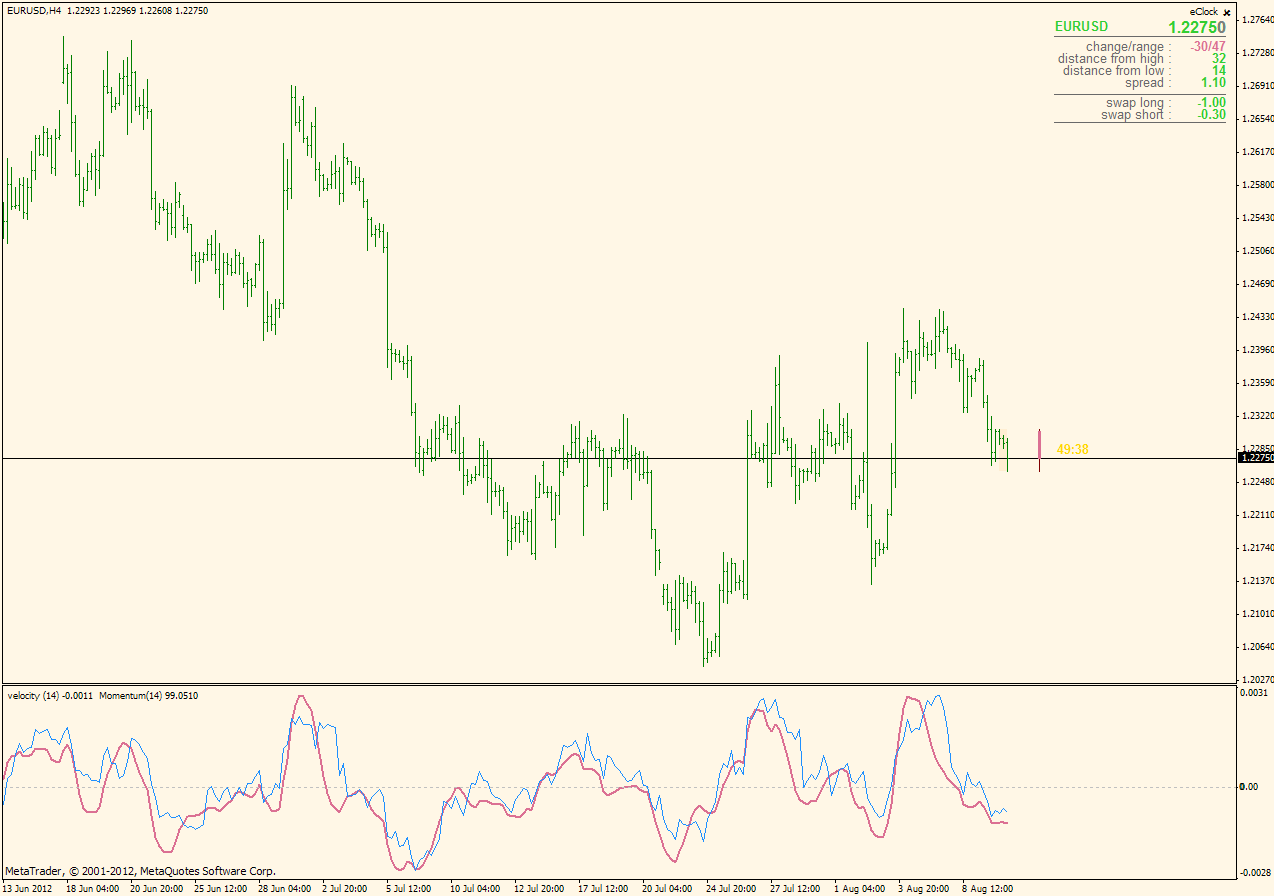PS: the way it is written, velocity can be easily calculated for any value, so in some sense, it covers the velocity and velocity on array in one single function

this settings to velocity

//OPEN PRICE

//HIGH PRICE

//CLOSE PRICE

//LOW PRICE

//MEDIAN PRICE

//TYPICAL PRICE

//WEIGHTED PRICE

if it is possible please create this.................thankyou255627

this settings to velocity

//OPEN PRICE

//HIGH PRICE

//CLOSE PRICE

//LOW PRICE

//MEDIAN PRICE

//TYPICAL PRICE

//WEIGHTED PRICE

if it is possible please create this.................thankyou

Here is a version with a drop-down list of those prices : velocity_2.mq4

Files:977

mrtools:
Talaat E, It's Brain Trend signal from here: https://www.mql5.com/en/forum/183798/page168

Thanks Mrtools, I missed this one so I didn't know about it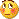186

mr.mladen sorry if i am asking too much please add smoothed to this indi with this settings and add (vertical lines include chart & oscillators) on zero line crossing.....sorry